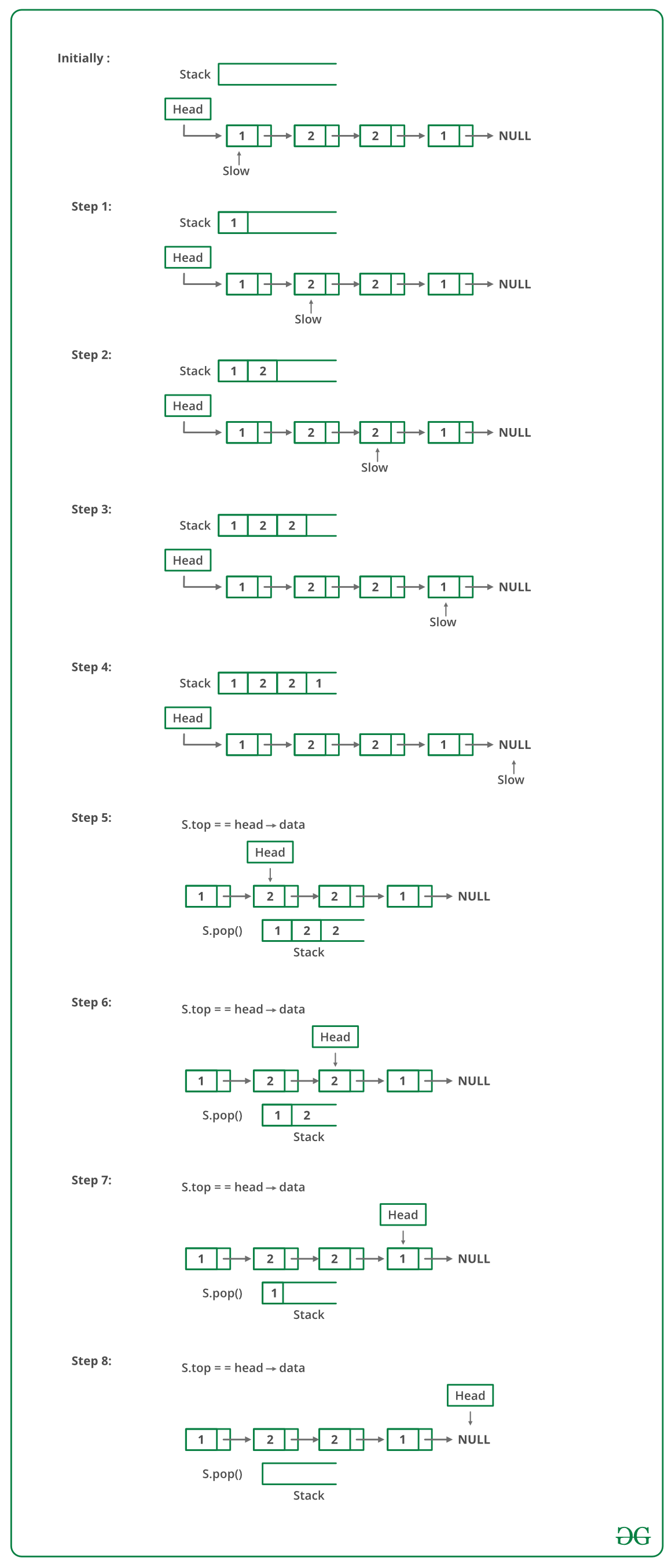GFG App
Open AppBrowser
Continue

# Javascript Program To Check If A Singly Linked List Is Palindrome

Given a singly linked list of characters, write a function that returns true if the given list is a palindrome, else false.METHOD 1 (Use a Stack)

• A simple solution is to use a stack of list nodes. This mainly involves three steps.
• Traverse the given list from head to tail and push every visited node to stack.
• Traverse the list again. For every visited node, pop a node from the stack and compare data of popped node with the currently visited node.
• If all nodes matched, then return true, else false.

Below image is a dry run of the above approach:Below is the implementation of the above approach :

## Javascript

 ``

Output:

` isPalindrome: true`

Time complexity: O(n), where n represents the length of the given linked list.

Auxiliary Space: O(n), for using a stack, where n represents the length of the given linked list.

METHOD 2 (By reversing the list):
This method takes O(n) time and O(1) extra space.
1) Get the middle of the linked list.
2) Reverse the second half of the linked list.
3) Check if the first half and second half are identical.
4) Construct the original linked list by reversing the second half again and attaching it back to the first half

To divide the list into two halves, method 2 of this post is used.

When a number of nodes are even, the first and second half contains exactly half nodes. The challenging thing in this method is to handle the case when the number of nodes is odd. We don’t want the middle node as part of the lists as we are going to compare them for equality. For odd cases, we use a separate variable ‘midnode’.

## Javascript

 ``

Output:

```a->NULL
Is Palindrome

b->a->NULL
Not Palindrome

a->b->a->NULL
Is Palindrome

c->a->b->a->NULL
Not Palindrome

a->c->a->b->a->NULL
Not Palindrome

b->a->c->a->b->a->NULL
Not Palindrome

a->b->a->c->a->b->a->NULL
Is Palindrome```

Time Complexity: O(n)
Auxiliary Space: O(1)

METHOD 3 (Using Recursion):
Use two pointers left and right. Move right and left using recursion and check for following in each recursive call.
1) Sub-list is a palindrome.
2) Value at current left and right are matching.

If both above conditions are true then return true.

The idea is to use function call stack as a container. Recursively traverse till the end of the list. When we return from the last NULL, we will be at the last node. The last node is to be compared with the first node of the list.

In order to access the first node of the list, we need the list head to be available in the last call of recursion. Hence, we pass head also to the recursive function. If they both match we need to compare (2, n-2) nodes. Again when recursion falls back to (n-2)nd node, we need a reference to 2nd node from the head. We advance the head pointer in the previous call, to refer to the next node in the list.
However, the trick is identifying a double-pointer. Passing a single pointer is as good as pass-by-value, and we will pass the same pointer again and again. We need to pass the address of the head pointer for reflecting the changes in parent recursive calls.
Thanks to Sharad Chandra for suggesting this approach.

## Javascript

 `

Output:

```a->NULL
Not Palindrome

b->a->NULL
Not Palindrome

a->b->a->NULL
Is Palindrome

c->a->b->a->NULL
Not Palindrome

a->c->a->b->a->NULL
Not Palindrome

b->a->c->a->b->a->NULL
Not Palindrome

a->b->a->c->a->b->a->NULL
Is Palindrome```

Time Complexity: O(n)
Auxiliary Space: O(n) if Function Call Stack size is considered, otherwise O(1).

Please refer complete article on Function to check if a singly linked list is palindrome for more details!

My Personal Notes arrow_drop_up